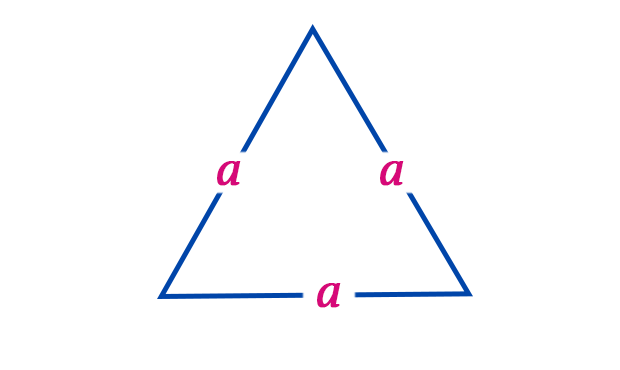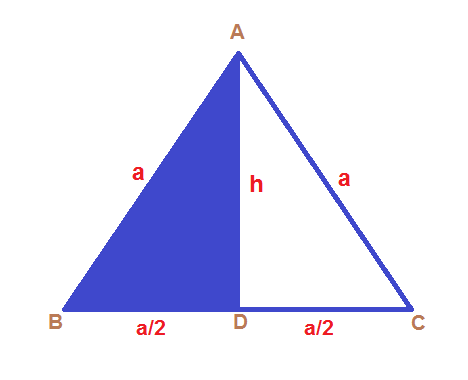# Area of An Equilateral Triangle• An equilateral triangle is a triangle in which all three sides are equal.
• Equilateral triangles are also equi-angular, which means, all three internal angles are also equal to each other and the only value possible is 60° each.
• The area of an equilateral triangle is basically the amount of space occupied by an equilateral triangle.
• Area of a triangle is measured in $unit^{2}$.
• In an equilateral triangle, the median, angle bisector and perpendicular are all the same and can be simply termed as the perpendicular bisector due to congruence conditions.
• A triangle is equilateral if and only if any three of the smaller triangles have either the same perimeter or the same inradius.
• A triangle is equilateral if and only if the circumcenters of any three of the smaller triangles have the same distance from the centroid.

Area of Equilateral Triangle Formula:  A = $\frac{\sqrt{3}}{4}$$a^{2} where, “a” denoted the sides of an Equilateral Triangle Proof:In the figure above, the sides of an equilateral triangle are equal to “a” units. We know that the area of Triangle is given by; A = $\frac{1}{2} \times base \times height$ To find the height, consider Triangle ABC, Applying Pythagoras Theorem we know, $AB^{2} = AD^{2}+BD^{2}$ $a^{2} = h^{2} + \left ( \frac{a}{2} \right )^{2}$ $h^{2} = a^{2} – \frac{a^{2}}{4}$ $h^{2} = \frac{3a^{2}}{4}$ $h = \frac{\sqrt{3}a}{2}$ Thus, we can calculate area by the basic equation, A = $\frac{1}{2} \times b \times h = \frac{1}{2} \times a \times \frac{\sqrt{3}a}{2}$ Therefore, A = $= \frac{\sqrt{3}a^{2}}{4} \;\; unit^{2}$ ### Lets work out a few examples:- Example 1: Find the area of an equilateral triangle whose side is 7 cm ? Solution: Given, Side of the equilateral triangle = a = 7 cm Area of an equilateral triangle = \frac{\sqrt{3}}{4} a^{2} = \frac{\sqrt{3}}{4}$$\times$$7^{2} cm^{2} = \frac{\sqrt{3}}{4}$$\times$49 $cm^{2}$

= 21.21762 $cm^{2}$

Example 2: Find the area of an equilateral triangle whose side is 28 cm ?

Solution:

Given,

Side of the equilateral triangle (a) = 28 cm

We know, Area of an equilateral triangle =  $\frac{\sqrt{3}}{4}$ $a^{2}$

= $\frac{\sqrt{3}}{4}$$\times$$28^{2}$ $cm^{2}$

= $\frac{\sqrt{3}}{4}$$\times$784 $cm^{2}$

= 339.48196 $cm^{2}$

For more, Formulas of triangles.